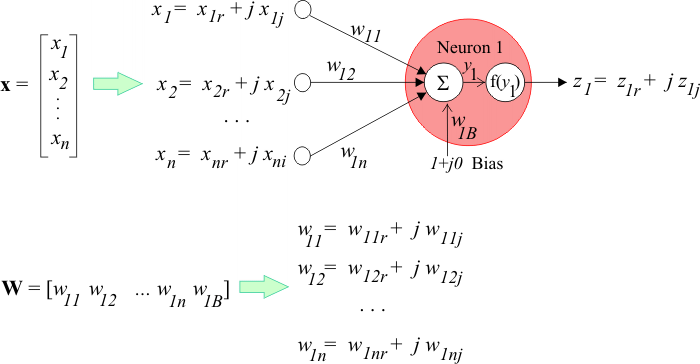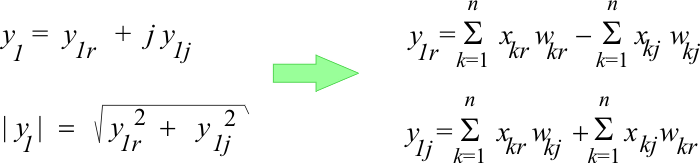# Introduction

## Complex Domain ANN

A complex-domain ANN is an ANN that has complex-domain: inputs, weights and outputs as shown in the figure below. They are used in problems where the data is organized in pairs. For instance, if the Fourier Transform is applied, the transformed data has a real and an imaginary part.Tip The sub-index r and j are used to represent the real and imaginary parts respectively of the complex number y. Thus, to compute the value of y in the previous figure, the algebra of complex numbers must be used as shown below.